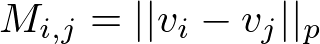# Feature Engineering with 3D Data Structures

Avvir deploys a wide range of statistical models in order to automatically detect deviations between buildings as-designed and as-built. But before we even begin fitting and testing these models, we have to decide how to represent our data.

Generally what we want to represent are points of interest in some metric space. In nearly all cases, we use 3-dimensional Euclidean distance, but the following techniques could be applied to any metric space. For instance, one might use the Mahalanobis distance, which warps space based on the measured covariance of a distribution of points, or the Manhattan distance, in order to prioritize alignment with the chosen coordinate system.

The notion of “points of interest” is also quite general. In the simplest case, this means an unordered set of 3-dimensional vectors with size n. This is colloquially called a “point cloud”, as it does not directly represent any relationships between points, just the points themselves:

In order to represent simple spatial relationships, we can compute the distances between each pair of points under our chosen distance metric, and put the results into an n-by-n symmetric matrix:Equation 2: a square symmetric matrix representing ℓ^p norms among a point cloud

We can take this a step further by partitioning the matrix to find local neighborhoods about each point, then binarizing to form a simple nearest-neighbor graph. This structure is the basis for several useful non-parametric predictive models, but has an important and particularly instructive drawback.

Since most pairs of points are too far apart to be considered strongly coupled, we generally set the binarization threshold to a neighborhood of less than a few dozen. This makes the adjacency matrix sparse, meaning most of its elements are null. For a point cloud on the order of 10 million points, this format can be very computationally inefficient. Practitioners typically use structures such as k-d trees and CSR matrices to reduce the time and space complexity of this structure from O(n²) to O(n), but the fact that the problem scales with the number of points rather than the extent of the space ought to catch our attention.

If we quantize space into 3-dimensional bins and keep track of the number of points in each bin, then the size of the structure is constant in the number of points. Furthermore, the spatial locality of the points is now baked into the structure. This makes it perfect for functions that operate on local neighborhoods of points independently, e.g. convolutional and morphologicaloperations. Such a data structure is commonly referred to as a “voxel grid” (vo- as in volume and -el as in element).